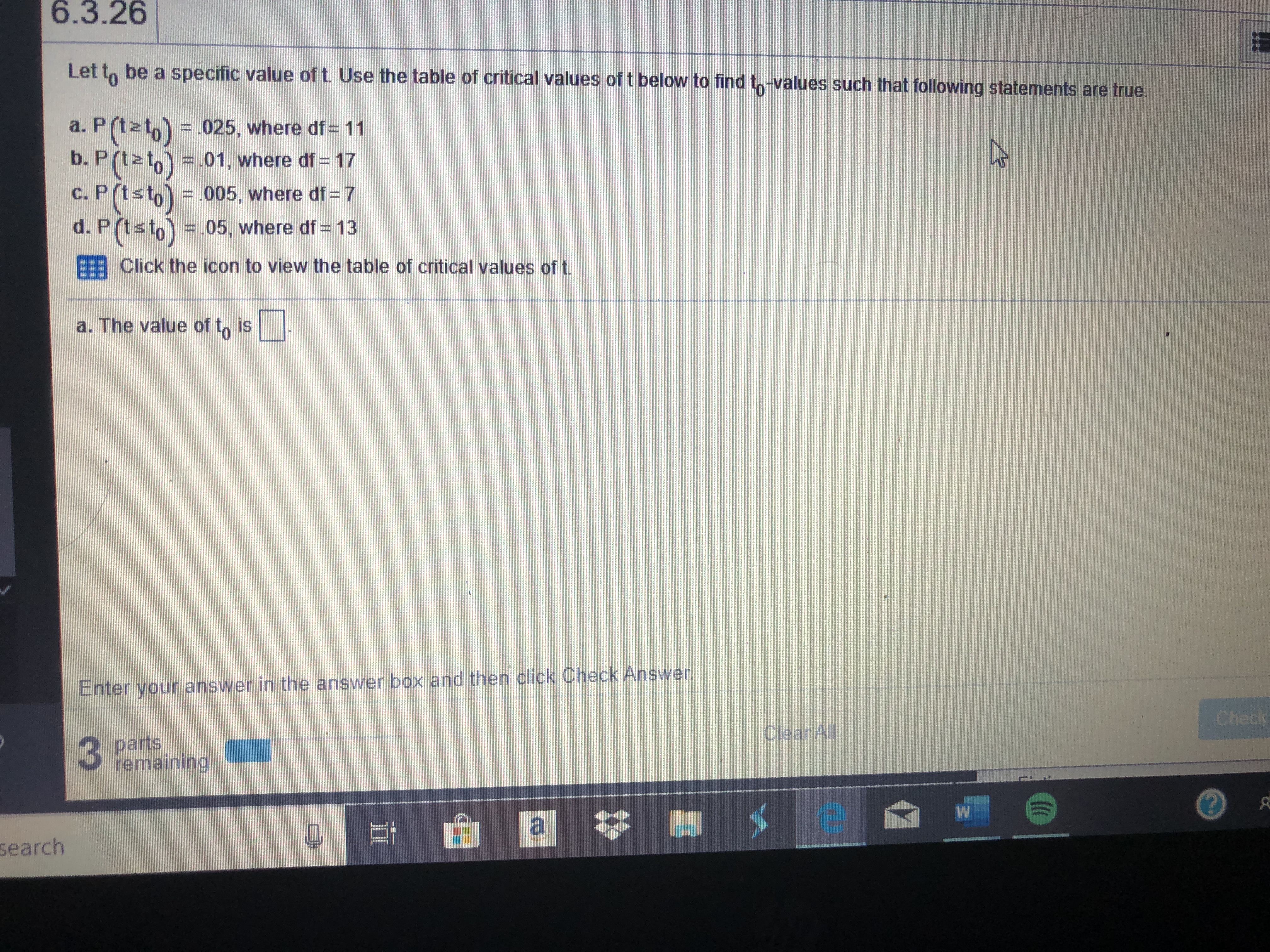# 6.3.26 Let to be a specific value of t. Use the table of critical values of t below to find to-values such that following statements are true. a. P(tzto) 025, where df= 11 b. P (tato) 01, where df 17 C. P(tsto) 005, where df= 7 d. P (tsto) 05, where df 13 E Click the icon to view the table of critical values of t. a. The value of to is Enter your answer in the answer box and then click Check Answer. Check Clear All parts remaining W a search

Questionhelp_outlineImage Transcriptionclose6.3.26 Let to be a specific value of t. Use the table of critical values of t below to find to-values such that following statements are true. a. P(tzto) 025, where df= 11 b. P (tato) 01, where df 17 C. P(tsto) 005, where df= 7 d. P (tsto) 05, where df 13 E Click the icon to view the table of critical values of t. a. The value of to is Enter your answer in the answer box and then click Check Answer. Check Clear All parts remaining W a search fullscreen

### Want to see this answer and more?

Experts are waiting 24/7 to provide step-by-step solutions in as fast as 30 minutes!*

*Response times vary by subject and question complexity. Median response time is 34 minutes and may be longer for new subjects.
Tagged in
MathStatistics